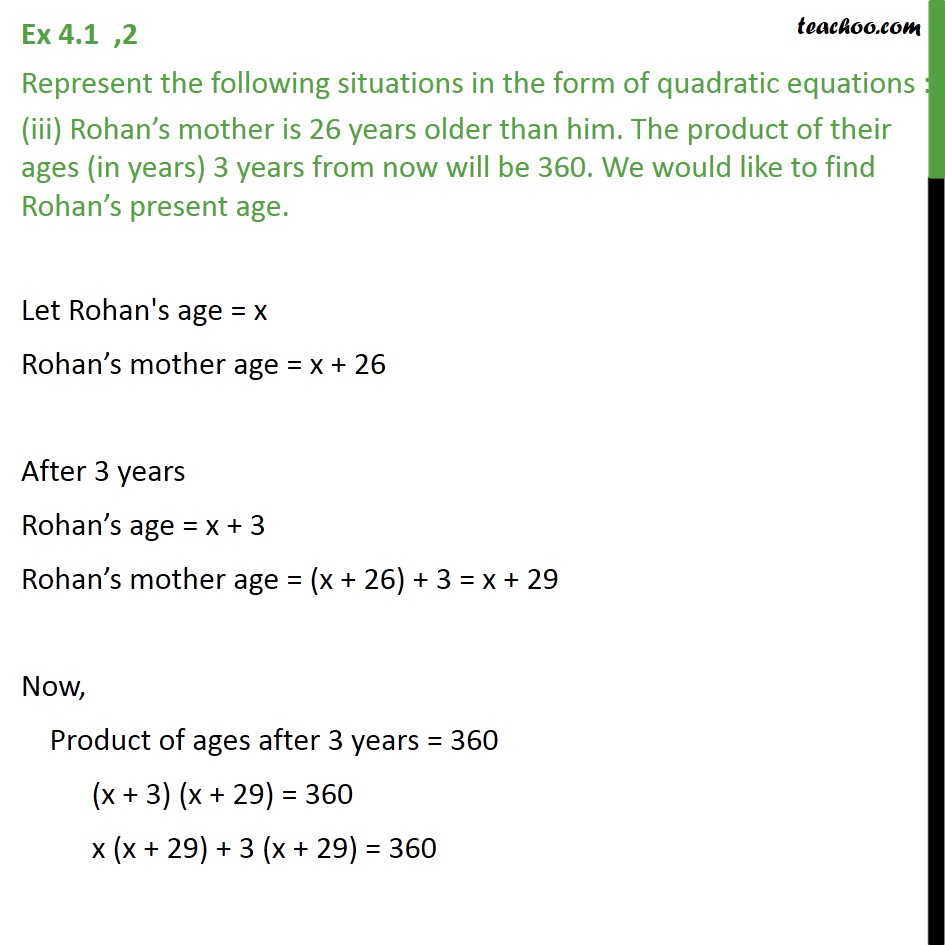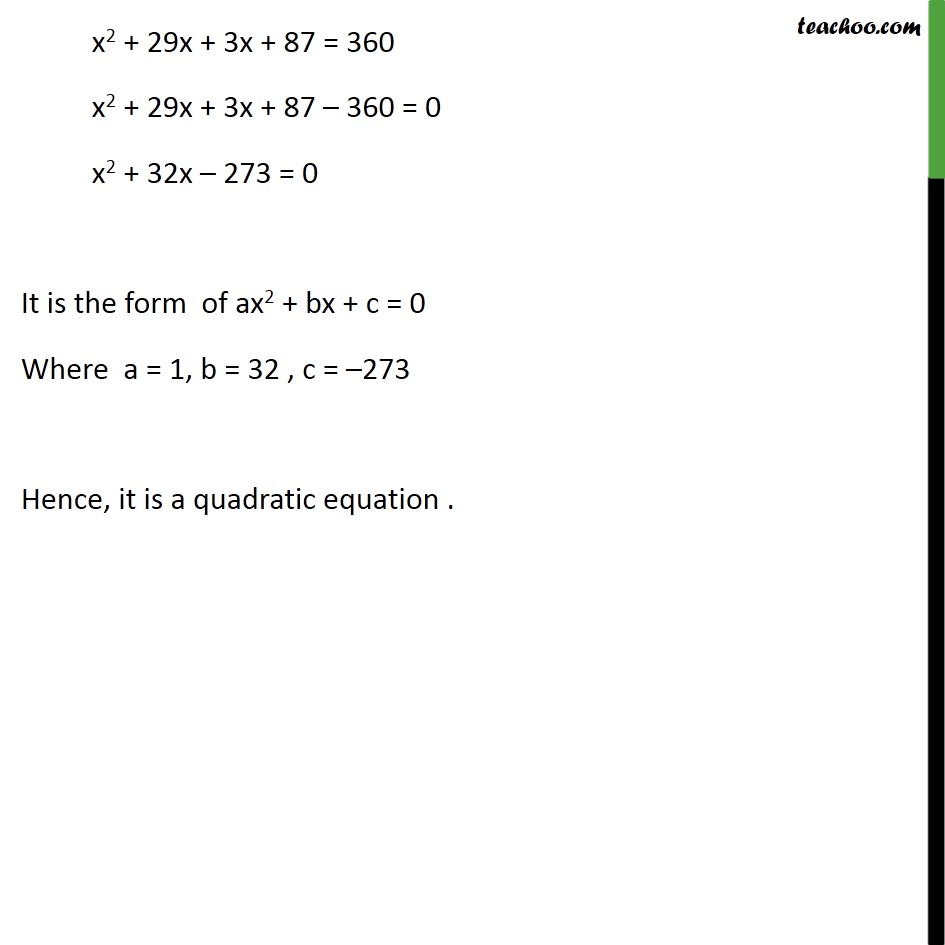Ex 4.1

Chapter 4 Class 10 Quadratic Equations
Serial order wiseLearn in your speed, with individual attention - Teachoo Maths 1-on-1 Class

### Transcript

Ex 4.1 ,2 Represent the following situations in the form of quadratic equations : (iii) Rohan s mother is 26 years older than him. The product of their ages (in years) 3 years from now will be 360. We would like to find Rohan s present age. Let Rohan's age = x Rohan s mother age = x + 26 After 3 years Rohan s age = x + 3 Rohan s mother age = (x + 26) + 3 = x + 29 Now, Product of ages after 3 years = 360 (x + 3) (x + 29) = 360 x (x + 29) + 3 (x + 29) = 360 x2 + 29x + 3x + 87 = 360 x2 + 29x + 3x + 87 360 = 0 x2 + 32x 273 = 0 It is the form of ax2 + bx + c = 0 Where a = 1, b = 32 , c = 273 Hence, it is a quadratic equation .